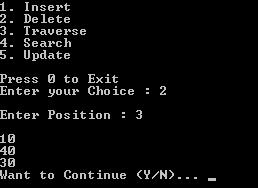# Linked List Types in Data Structure

Following are the types of Linked List

• Each node has a single link to another node is called Singly Linked List.
• Singly Linked List does not store any pointer any reference to the previous node.
• Each node stores the contents of the node and a reference to the next node in the list.
• In a singly linked list, last node has a pointer which indicates that it is the last node. It requires a reference to the first node to store a single linked list.
• It has two successive nodes linked together in linear way and contains address of the next node to be followed.
• It has successor and predecessor. First node does not have predecessor while last node does not have successor. Last node have successor reference as NULL.
• It has only single link for the next node.
• In this type of linked list, only forward sequential movement is possible, no direct access is allowed.

•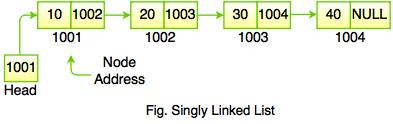• In the above figure, the address of the first node is always store in a reference node known as Head or Front. Reference part of the last node must be null.

#### Example: Program to perform various operations on Singly Linked List

#include<stdio.h>
#include<stdlib.h>
typedef struct Node
{
int data;
struct Node *next;
}node;
void insert(node *pointer, int data)
{
/* Iterate through the list till we encounter the last node.*/
while(pointer->next!=NULL)
{
pointer = pointer -> next;
}
/* Allocate memory for the new node and put data in it.*/
pointer->next = (node *)malloc(sizeof(node));
pointer = pointer->next;
pointer->data = data;
pointer->next = NULL;
}
int find(node *pointer, int key)
{
pointer =  pointer -> next; //First node is dummy node.
/* Iterate through the entire linked list and search for the key. */
while(pointer!=NULL)
{
if(pointer->data == key) //key is found.
{
return 1;
}
pointer = pointer -> next;//Search in the next node.
}
return 0;
}
void delete(node *pointer, int data)
{
/* Go to the node for which the node next to it has to be deleted */
while(pointer->next!=NULL && (pointer->next)->data != data)
{
pointer = pointer -> next;
}
if(pointer->next==NULL)
{
printf("Element %d is not present in the list\n",data);
return;
}
/* Now pointer points to a node and the node next to it has to be removed */
node *temp;
temp = pointer -> next;
/*temp points to the node which has to be removed*/
pointer->next = temp->next;
/*We removed the node which is next to the pointer (which is also temp) */
free(temp);
/* Beacuse we deleted the node, we no longer require the memory used for it .
free() will deallocate the memory.
*/
return;
}
void print(node *pointer)
{
if(pointer==NULL)
{
return;
}
printf("%d ",pointer->data);
print(pointer->next);
}
int main()
{
/* start always points to the first node of the linked list.
temp is used to point to the last node of the linked list.*/
node *start,*temp;
start = (node *)malloc(sizeof(node));
temp = start;
temp -> next = NULL;
/* Here in this code, we take the first node as a dummy node.
The first node does not contain data, but it used because to avoid handling special cases in insert and delete functions. */
printf("1. Insert\n");
printf("2. Delete\n");
printf("3. Print\n");
printf("4. Find\n");
while(1)
{
int query;
scanf("%d",&query);
if(query==1)
{
int data;
printf("Enter the element: ");
scanf("%d",&data);
insert(start,data);
printf("Press 3 for display: ");
}
else if(query==2)
{
int data;
printf("Delete the element: ");
scanf("%d",&data);
delete(start,data);
printf("Press 3 for display: ");
}
else if(query==3)
{
printf("The list is ");
print(start->next);
printf("\n");
}
else if(query==4)
{
int data;
printf("Find the element: ");
scanf("%d",&data);
int status = find(start,data);
if(status)
{
printf("Element Found\n");
}
else
{

}
}
}
}

Output: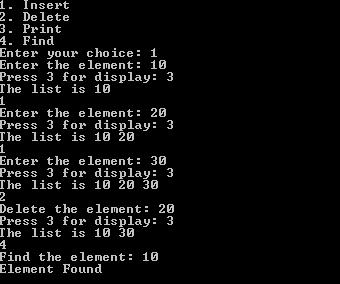• Doubly linked list is a sequence of elements in which every node has link to its previous node and next node.
• Traversing can be done in both directions and displays the contents in the whole list.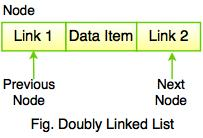In the above figure, Link1 field stores the address of the previous node and Link2 field stores the address of the next node. The Data Item field stores the actual value of that node. If we insert a data into the linked list, it will be look like as follows: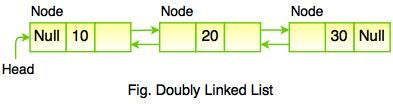Important Note:
First node is always pointed by head. In doubly linked list, previous field of the first node is always NULL (it must be NULL) and the next field of the last must be NULL.

In the above figure we see that, doubly linked list contains three fields. In this, link of two nodes allow traversal of the list in either direction. There is no need to traverse the list to find the previous node. We can traverse from head to tail as well as tail to head.

• Doubly linked list can be traversed in both forward and backward directions.
• To delete a node in singly linked list, the previous node is required, while in doubly linked list, we can get the previous node using previous pointer.
• It is very convenient than singly linked list. Doubly linked list maintains the links for bidirectional traversing.

• In doubly linked list, each node requires extra space for previous pointer.
• All operations such as Insert, Delete, Traverse etc. require extra previous pointer to be maintained.

#### Example: Program to perform various operations on Doubly Linked List

#include<stdio.h>
#include<stdlib.h>

typedef struct Node
{
int data;
struct Node *next;
struct Node *prev;
}node;

void insert(node *pointer, int data)
{
/* Iterate through the list till we encounter the last node.*/
while(pointer->next!=NULL)
{
pointer = pointer -> next;
}
/* Allocate memory for the new node and put data in it.*/
pointer->next = (node *)malloc(sizeof(node));
(pointer->next)->prev = pointer;
pointer = pointer->next;
pointer->data = data;
pointer->next = NULL;
}

int find(node *pointer, int key)
{
pointer =  pointer -> next; //First node is dummy node.
/* Iterate through the entire linked list and search for the key. */
while(pointer!=NULL)
{
if(pointer->data == key) //key is found.
{
return 1;
}
pointer = pointer -> next;//Search in the next node.
}
return 0;
}

void delete(node *pointer, int data)
{
/* Go to the node for which the node next to it has to be deleted */
while(pointer->next!=NULL && (pointer->next)->data != data)
{
pointer = pointer -> next;
}
if(pointer->next==NULL)
{
printf("Element %d is not present in the list\n",data);
return;
}
/* Now pointer points to a node and the node next to it has to be removed */

node *temp;
temp = pointer -> next;

/*temp points to the node which has to be removed*/
pointer->next = temp->next;
temp->prev =  pointer;
/*We removed the node which is next to the pointer (which is also temp) */
free(temp);
/* Beacuse we deleted the node, we no longer require the memory used for it .
free() will deallocate the memory. */
return;
}
void print(node *pointer)
{
if(pointer==NULL)
{
return;
}
printf("%d ",pointer->data);
print(pointer->next);
}

int main()
{
/* start always points to the first node of the linked list.
temp is used to point to the last node of the linked list.*/
node *start,*temp;
start = (node *)malloc(sizeof(node));
temp = start;
temp -> next = NULL;
temp -> prev = NULL;
/* Here in this code, we take the first node as a dummy node.
The first node does not contain data, but it used because to avoid handling special cases
in insert and delete functions.
*/
printf("1. Insert\n");
printf("2. Delete\n");
printf("3. Print\n");
printf("4. Find\n");
printf("Enter Choice: ");
while(1)
{
int query;
scanf("%d",&query);
if(query==1)
{
int data;
printf("Enter the element: ");
scanf("%d",&data);
insert(start,data);
printf("Enter 3 for display: ");
}
else if(query==2)
{
int data;
printf("Delete the element: ");
scanf("%d",&data);
delete(start,data);
printf("Enter 3 for display: ");
}
else if(query==3)
{
printf("The list is: ");
print(start->next);
printf("\n");
//main();
}
else if(query==4)
{
int data;
printf("Find the element: ");
scanf("%d",&data);
int status = find(start,data);
if(status)
{
printf("Element Found\n");
}
else
{

}
}
}
}

Output: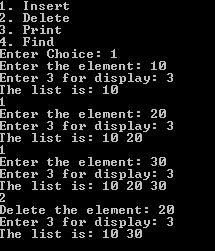• Circular linked list is similar to singly linked list. The only difference is that in circular linked list, the last node points to the first node in the list.
• It is a sequence of elements in which every element has link to its next element in the sequence and has a link to the first element in the sequence.

•• In the above figure we see that, each node points to its next node in the sequence but the last node points to the first node in the list. The previous element stores the address of the next element and the last element stores the address of the starting element. It forms a circular chain because the element points to each other in a circular way.
• In circular linked list, the memory can be allocated when it is required because it has a dynamic size.
• Circular linked list is used in personal computers, where multiple applications are running. The operating system provides a fixed time slot for all running applications and the running applications are kept in a circular linked list until all the applications are completed. This is a real life example of circular linked list.
• We can insert elements anywhere in circular linked list, but in the array we cannot insert elements anywhere in the list because it is in the contiguous memory.

## 4. Doubly Circular Linked List

• Doubly circular linked list is a linked data structure which consists of a set of sequentially linked records called nodes.
• Doubly circular linked list can be conceptualized as two singly linked lists formed from the same data items, but in opposite sequential orders.

•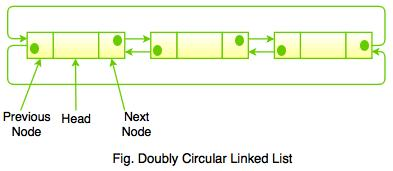• The above diagram represents the basic structure of Doubly Circular Linked List. In doubly circular linked list, the previous link of the first node points to the last node and the next link of the last node points to the first node.
• In doubly circular linked list, each node contains two fields called links used to represent references to the previous and the next node in the sequence of nodes.

#### Example: Program to perform various operations on Doubly Circular Linked List

#include<stdio.h>
#include<conio.h>
#include<malloc.h>
#include<stdlib.h>

int data;
struct DCLL
{
int info;
struct DCLL *rptr, *lptr;
}*start = NULL, *temp, *neww, *prev, *end = NULL;

void ins_beg_dcll();
void ins_end_dcll();
void del_beg_dcll();
void del_end_dcll();
void create_dcll();
void travers();
void insert();
void delet();
void search();
void update();

void main()
{
int choice;
char ch, ch2 = 'y';
do
{
if (start == NULL)
{
do
{
// clrscr();
printf("\nError : Linked LIst is Empty Want to Create (Y/N).. ");
fflush(stdin);
scanf("%c", &ch);
if (ch == 'n' || ch == 'N')
{
exit(0);
}
else if (ch == 'y' || ch == 'Y')
{
create_dcll();
break;
}
else
{
printf("\nError : Invalid Choice");
}
} while (ch == 'y' || ch == 'Y');
}
else
{
// clrscr();
printf("\n1. Insert");
printf("\n2. Delete");
printf("\n3. Traverse");
printf("\n4. Search");
printf("\n5. Update");
printf("\n\nPress 0 to Exit");
scanf("%d", &choice);

switch (choice)
{
case 0:
exit(0);
break;
case 1:
insert();
travers();
break;
case 2:
delet();
travers();
break;
case 3:
travers();
break;
case 4:
search();
break;
case 5:
update();
break;
default:
printf("\nError : Invalid Choice. \n");
}
printf("\nWant to Continue (Y/N)... ");
fflush(stdin);
scanf("%c", &ch2);
}

}while (ch2 == 'y' || ch2 == 'Y');
}
void create_dcll()
{
char ch;
do
{
//clrscr();
printf("\nEnter Node Info");
printf("\n\nEnter Info : ");
scanf("%d", &data);
temp = (struct DCLL *)malloc(sizeof(struct DCLL));
temp->info = data;
temp->rptr = start;
temp->lptr = start;

if (start == NULL)
{
start = temp;
end = temp;
start->rptr = start;
start->lptr = start;
}
else
{
temp->lptr = end;
end->rptr = temp;
temp->rptr = start;
start->lptr = temp;
end = temp;
}
printf("\nDo you want to insert another Node (Y/N)... ");
fflush(stdin);
scanf("%c", &ch);
} while (ch == 'y' || ch == 'Y');
}
void ins_beg_dcll()
{
neww->rptr = start;
start->lptr = neww;
neww->lptr = end;
end->rptr = neww;
start = neww;
}
void ins_end_dcll()
{
neww->lptr = end;
end->rptr = neww;
neww->rptr = start;
start->lptr = neww;
end = neww;
}
void travers()
{
temp = start;
if (start == NULL)
{
printf("\nError : Linked list is Empty");
}
else
{
if (start == end)
{
printf("\n%d", temp->info);
}
else
{
printf("\n%d", temp->info);
do
{
temp = temp->rptr;
printf("\n%d", temp->info);
} while (temp != end);
}
}
}
void insert()
{
int pos, count = 1;
printf("\nEnter Position : ");
scanf("%d", &pos);
printf("\nEnter Info :");
scanf("%d", &data);

neww = (struct DCLL *)malloc(sizeof(struct DCLL));
neww->info = data;
temp = start;

if (pos == 1)
{
ins_beg_dcll();
}
else
{
while (count != pos - 1 && temp->rptr != start)
{
count++;
temp = temp->rptr;
}
if (temp->rptr == start && count < pos - 1)
{
printf("\nError : Invalid Position...");
}
else if (temp->rptr == start && count == pos - 1)
{
ins_end_dcll();
}
else
{
neww->rptr = temp->rptr;
neww->lptr = temp;
temp->rptr->lptr = neww;
temp->rptr = neww;
}
}
}
void delet()
{
int pos, count = 1;
printf("\nEnter Position : ");
scanf("%d", &pos);
temp = start;
if (pos == 1)
{
del_beg_dcll();
}
else
{
while (count != pos && temp != end)
{
prev = temp;
temp = temp->rptr;
count++;
}
if (temp == end && count < pos - 1)
{
printf("\nError : Invalid Position.");
}
else if (temp == end && count == pos)
{
del_end_dcll();
}
else
{
prev->rptr = temp->rptr;
temp->rptr->lptr = prev;
free(temp);
}
}
}
void del_beg_dcll()
{
start = start->rptr;
start->lptr = end;
end->rptr = start;
free(temp);
}
void del_end_dcll()
{
end = end->lptr;
end->rptr = start;
start->lptr = end;
free(temp);
}
void search()
{
int src, count = 1;
printf("\nEnter Info : ");
scanf("%d", &src);
temp = start;
do
{
prev = temp;
if (prev->info == src)
{
printf("\nSearch Value found at %d Position.", count);
break;
}
count++;
temp = temp->rptr;
} while (prev != end);
if (temp == start)
{
printf("\nError : Search value doesn't exists.");
}
}
void update()
{
int pos, count = 1, dt;
printf("\nEnter Position : ");
scanf("%d", &pos);
printf("\nEnter New Info : ");
scanf("%d", &dt);
temp = prev = start;
do
{
if (count == pos && prev != end)
{
prev->info = dt;
break;
}
prev = temp;
temp = temp->rptr;
count++;
} while (prev != end);

if (temp == start)
{
printf("\nError : Invalid Choice ");
}
}

Output:

1. Create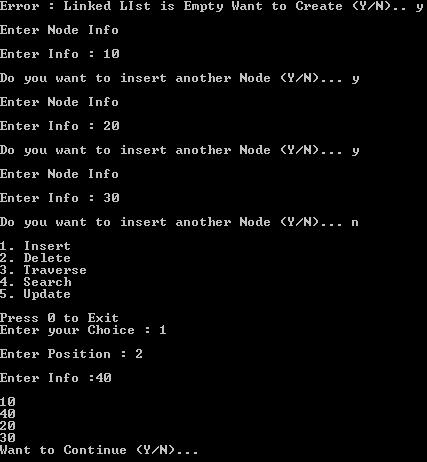2. Search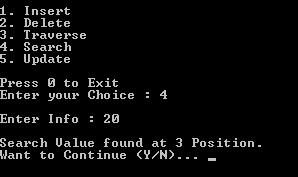3. Delete